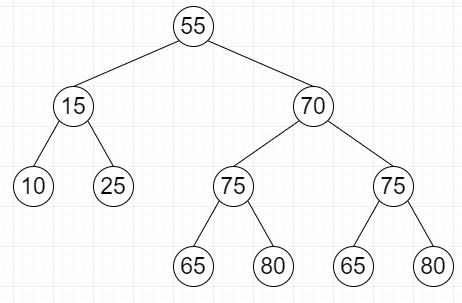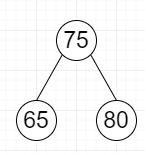# Find largest subtree having identical left and right subtrees in Python

Suppose we have a binary tree; we have to find the largest subtree having identical left and right subtree. Preferred time complexity is O(n).

So, if the input is likethen the output will beTo solve this, we will follow these steps −

• Define a function solve() . This will take root, encode, maxSize, maxNode

• if root is None, then

• return 0

• left_list := list with a blank string

• right_list := list with a blank string

• ls := solve(root.left, left_list, maxSize, maxNode)

• rs := solve(root.right, right_list, maxSize, maxNode)

• size := ls + rs + 1

• if left_list is same as right_list, then

• if size > maxSize, then

• maxSize := size

• maxNode := root

• encode := encode concatenate "|" concatenate left_list concatenate "|"

• encode := encode concatenate "|" concatenate str(root.data) concatenate "|"

• encode := encode concatenate "|" concatenate right_list concatenate "|"

• return size

• From the main method, do the following −

• maximum := 

• encode := list with a blank string

• solve(node, encode, maximum, maxNode)

• return maximum

## Example

Let us see the following implementation to get better understanding −

Live Demo

class TreeNode:
def __init__(self, data):
self.data = data
self.left = self.right = None
def solve(root, encode, maxSize, maxNode):
if (root == None):
return 0
left_list = [""]
right_list = [""]
ls = solve(root.left, left_list, maxSize, maxNode)
rs = solve(root.right, right_list, maxSize, maxNode)
size = ls + rs + 1
if (left_list == right_list):
if (size > maxSize):
maxSize = size
maxNode = root
encode = encode + "|" + left_list + "|"
encode = encode + "|" + str(root.data) + "|"
encode = encode + "|" + right_list + "|"

return size

def largestSubtree(node, maxNode):
maximum = 
encode = [""]
solve(node, encode, maximum,maxNode)
return maximum
root = TreeNode(55)
root.left = TreeNode(15)
root.right = TreeNode(70)
root.left.left = TreeNode(10)
root.left.right = TreeNode(25)
root.right.left = TreeNode(75)
root.right.left.left = TreeNode(65)
root.right.left.right = TreeNode(80)
root.right.right = TreeNode(75)
root.right.right.left = TreeNode(65)
root.right.right.right = TreeNode(80)
maxNode = [None]
maximum = largestSubtree(root, maxNode)
print("Root of largest sub-tree",maxNode.data)
print("and its size is ", maximum)

## Input

root = TreeNode(55)
root.left = TreeNode(15)
root.right = TreeNode(70)
root.left.left = TreeNode(10)
root.left.right = TreeNode(25)
root.right.left = TreeNode(75)
root.right.left.left = TreeNode(65)
root.right.left.right = TreeNode(80)
root.right.right = TreeNode(75)
root.right.right.left = TreeNode(65)
root.right.right.right = TreeNode(80)

## Output

Root of largest sub-tree 70
and its size is 

Updated on: 25-Aug-2020

62 Views